International
Tables for
Crystallography
Volume F
Crystallography of biological macromolecules
Edited by M. G. Rossmann and E. Arnold

International Tables for Crystallography (2006). Vol. F, ch. 15.2, p. 325   | 1 | 2 |

Section 15.2.2. Model bias: importance of phase

aDepartment of Haematology, University of Cambridge, Wellcome Trust Centre for Molecular Mechanisms in Disease, CIMR, Wellcome Trust/MRC Building, Hills Road, Cambridge CB2 2XY, England
Correspondence e-mail: rjr27@cam.ac.uk

15.2.2. Model bias: importance of phase

| top | pdf |

Dramatic illustrations of the importance of the phase have been published. For instance, Ramachandran & Srinivasan (1961)calculated an electron-density map using phases from one structure and amplitudes from another. In this map there are peaks at the positions of the atoms in the structure that contributed the phase information, but not in the structure that contributed the amplitudes. Similar calculations with two-dimensional Fourier transforms of photographs (Oppenheim & Lim, 1981; Read, 1997) show that the phases of one completely overwhelm the amplitudes of the other.

These examples, though dramatic, are not completely representative of the normal situation, where the structure contributing the phases is partially or even nearly correct. Nonetheless, model phases always contribute bias, so that the resulting map tends to bear too close a resemblance to the model.

15.2.2.1. Parseval's theorem

| top | pdf |

The importance of the phase can be understood most easily in terms of Parseval's theorem, a result that is important to the understanding of many aspects of the Fourier transform and its use in crystallography. Parseval's theorem states that the mean-square value of the variable on one side of a Fourier transform is proportional to the mean-square value of the variable on the other side. Since the Fourier transform is additive, Parseval's theorem also applies to sums or differences.

Ifandare, for instance, the true electron density and the electron density of the model, respectively, Parseval's theorem tells us that the r.m.s. error in the electron density is proportional to the r.m.s. error in the structure factor. (The structure-factor error is a vector error in the complex plane.)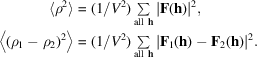This understanding of error in electron-density maps explains why the phase is much more important than the amplitude in determining the appearance of an electron-density map. As illustrated in Fig. 15.2.2.1,a random choice of phase (from a uniform distribution of all possible phases) will generally give a larger error in the complex plane than a random choice of amplitude [from a Wilson (1949)distribution of amplitudes].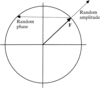Figure 15.2.2.1 | top | pdf |Schematic illustration of the relative errors introduced by a random choice of phase or a random choice of amplitude. The example has been constructed to represent the r.m.s. errors introduced by randomization (computed by averages over the Wilson distribution). Phase randomization will introduce r.m.s. errors of(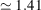) times the r.m.s. structure-factor amplitude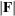. By comparison, map coefficients weighted by figures of merit of zero would have r.m.s. errors equal to the r.m.s., so a featureless map would be more accurate than a random-phase map. Amplitude randomization will introduce r.m.s. errors of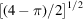(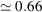) times the r.m.s., so a map computed with random amplitudes will be closer to the true map than a featureless map.

References

Oppenheim, A. V. & Lim, J. S. (1981). The importance of phase in signals. Proc. IEEE, 69, 529–541.Google Scholar
Ramachandran, G. N. & Srinivasan, R. (1961). An apparent paradox in crystal structure analysis. Nature (London), 190, 159–161.Google Scholar
Read, R. J. (1997). Model phases: probabilities and bias. Methods Enzymol. 277, 110–128.Google Scholar
Wilson, A. J. C. (1949). The probability distribution of X-ray intensities. Acta Cryst. 2, 318–321.Google Scholar